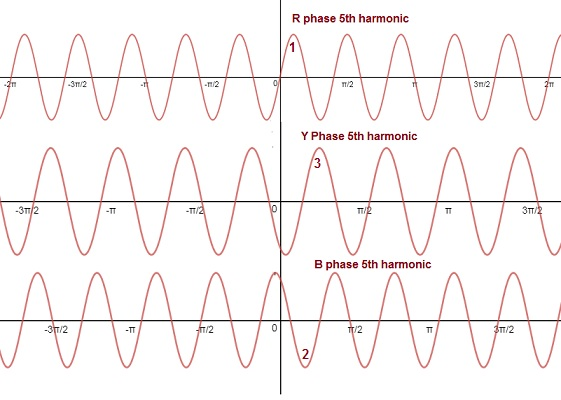# Triplen Harmonics – Why are they in phase?

### Definition:

Triplen harmonics are the odd integral multiple of third harmonic wave. If the frequency of third harmonic wave is ω then frequency of triplen harmonic wave will be 3nω, where n = 3,5,9,…These waves posses a special characteristics and hence it becomes important for the study and analysis of power system. The amazing property of triplen harmonics is that they are in time phase in the three output phase of alternator.

### Why triplen harmonics are in phase?

Let us consider a three phase alternator having identical phase windings i.e. R, Y and B in which harmonics are produced. Let the three phase emf are VR, VY and VB. These emf can be represented as below.

VR = E1msin(ωt+Ø1) + E3msin(3ωt+Ø3) + E5msin(5ωt+Ø5) +…….

VY = E1msin(ωt-2π/3+Ø1) + E3msin[3(ωt-2π/3)+Ø3] + E5msin[5(ωt-2π/3)+Ø5] +…….

VB = E1msin(ωt-4π/3+Ø1) + E3msin[3(ωt-4π/3)+Ø3] + E5msin[5(ωt-4π/3)+Ø5] +…….

Let us simplify each of these phase voltages.

VR = E1msin(ωt+Ø1) + E3msin(3ωt+Ø3) + E5msin(5ωt+Ø5) +…….

VY = E1msin(ωt-2π/3+Ø1) + E3msin[3(ωt-2π/3)+Ø3] + E5msin[5(ωt-2π/3)+Ø5] +…….

= E1msin(ωt-2π/3+Ø1) + E3msin[(3ωt-2π)+Ø3] + E5msin[(5ωt-10π/3)+Ø5] +…….

= E1msin(ωt-2π/3+Ø1) + E3msin(3ωt+Ø3) + E5msin(5ωt-4π/3+Ø5) +…….

Now,

VB = E1msin(ωt-4π/3+Ø1) + E3msin[3(ωt-4π/3)+Ø3] + E5msin[5(ωt-4π/3)+Ø5] +…….

= E1msin(ωt-4π/3+Ø1) + E3msin[(3ωt-4π)+Ø3] + E5msin[(5ωt-20π/3)+Ø5] +…….

= E1msin(ωt-4π/3+Ø1) + E3msin(3ωt+Ø3) + E5msin(5ωt-2π/3+Ø5) +…….

Carefully observe the above three expressions for individual phase voltages. It can easily be seen that, all the three phase voltages only contain odd harmonics. They do not contain any even harmonics. This is because “alternator phase voltages do not have even harmonics”. For detail please read “Why Output Voltage of Alternator can’t have Even Harmonics?

Also, the third harmonic component in each of the VR, VY and VB are same in i.e. E3msin(3ωt+Ø3). Thus we can say that, all triplen harmonics in the three phases are in time phase.

The fifth harmonic components in emf wave are below.

5th Harmonic in VR = E5msin(5ωt+Ø5)

5th Harmonic in VY = E5msin(5ωt-4π/3+Ø5)

5th Harmonic in VB = E5msin(5ωt-2π/3+Ø5)

But the fundamental components in emf wave are

Fundamental Component in VR = E1msin(ωt+Ø1)

Fundamental Component in VY = E1msin(ωt-2π/3+Ø1)

Fundamental Component in VB = E1msin(ωt-4π/3+Ø1)

By comparison of 5th harmonic components with fundamental, we can say that phase sequence of 5th harmonic are opposite to the fundamental. This mean the phase sequence will be R, B and Y for fifth harmonics. Hence, they have negative phase sequence. The waveform is shown below.It is clear from the above waveform that first R phase gets maximum followed by B and Y phase. Therefore their phase sequence is R, B and Y.

### Conclusion:

• All triplen harmonics i.e. 3rd, 9th, 15th etc. are in phase.
• The 7th, 13th, 19th etc. have positive phase rotation of R, Y and B.
• The 5th, 11th, 17th etc. have negative phase sequence of R, B and Y.

This site uses Akismet to reduce spam. Learn how your comment data is processed.